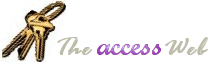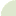Home  |   French  |   About  |   Search  | mvps.org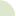What's New Table Of Contents Credits Netiquette 10 Commandments  Bugs Tables Queries Forms Reports Modules APIs Strings Date/Time General Downloads Resources Search Feedback mvps.org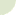Modules: Determining the number of dimensions for an array

Author(s)
Lyle FairfieldThere is no direct way to determine the number of dimensions in an Array in VBA.  This is not the same as the value returned by UBound which lets us find out the largest available subscript for the indicated dimension.

One way to determine the number of dimensions is to start with the LBound value and keep increasing  the dimension value until an error occurs.  The number of dimensions is one less than the value where the error occurred.  This technique is explained by this Knowledge Base  article.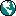XL: Determining the Number of Dimensions in an Array Variable Article ID: 152288
A more complicated way would be to get to the SAFEARRAY that VBA creates internally for the actual array and then use RTLMoveMemory API function to determine the number of dimensions.

A safer and easier way is to use this code.

```'************ Code Start **********
'This code was originally written by Lyle Fairfield
'It is not to be altered or distributed,
'except as part of an application.
'You are free to use it in any application,
'provided the copyright notice is left unchanged.
'
'Code Courtesy of
'Lyle Fairfield
'
Function ElementCount(b As Variant) As Long
Dim v As Variant, z As Long
For Each v In b
z = z + 1
Next v
Do
ElementCount = ElementCount + 1
z = z / (UBound(b, ElementCount) - LBound(b, ElementCount) + 1)
Loop Until z = 1
End Function

Sub testArray()
Dim a(3 To 9, 4 To 7, 0, 1 To 12) As Variant, b As Variant
Dim varReturn As Long
b = a
varReturn = fDummy(b)
MsgBox varReturn
End Sub

Function fDummy(b As Variant) As Long
fDummy = ElementCount(b)
End Function
'********** Code End ***********```© 1998-2010, Dev Ashish & Arvin Meyer, All rights reserved. Optimized for Microsoft Internet Explorer